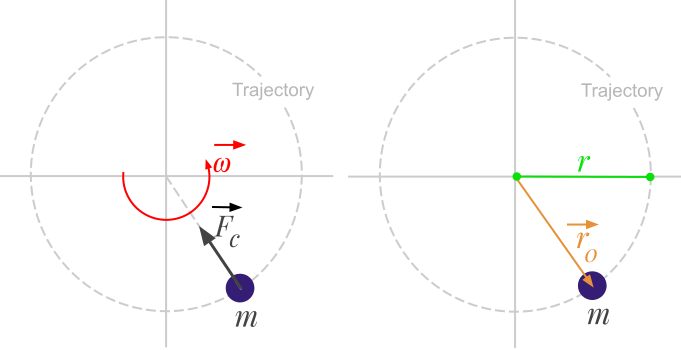Question #440

# What is the formula of the centripetal force based on angular velocity ?

Merged questionsThe centripetal force can be calculated with the following formula:

﻿﻿

The previous equation can be generalized in vector form:

﻿﻿

Where:

• ﻿﻿ is the centripetal force expressed in Newton (﻿﻿)
• ﻿﻿ is the body mass expressed in kilograms (﻿﻿)
• ﻿﻿ is the angular velocity of the body expressed in radians per second (﻿﻿)
• ﻿﻿ is the angular velocity vector
• ﻿﻿ is the radius of the circular path expressed in meters (﻿﻿)
• ﻿﻿ is a radius vector attached to the center of the circular path, in the direction of the body.
3 events in history
Question by Alphonsio 12/11/2020 at 06:23:46 PM
How to calculate the centripetal force based on angular velocity ?
Answer by Alphonsio 12/11/2020 at 06:22:59 PMThe centripetal force can be calculated with the following formula:

﻿﻿

The previous equation can be generalized in vector form:

﻿﻿

Where:

• ﻿﻿ is the centripetal force expressed in Newton (﻿﻿)
• ﻿﻿ is the body mass expressed in kilograms (﻿﻿)
• ﻿﻿ is the angular velocity of the body expressed in radians per second (﻿﻿)
• ﻿﻿ is the angular velocity vector
• ﻿﻿ is the radius of the circular path expressed in meters (﻿﻿)
• ﻿﻿ is a radius vector attached to the center of the circular path, in the direction of the body.
Question by Alphonsio 12/11/2020 at 06:21:17 PM
What is the formula of the centripetal force based on angular velocity ?

Icons proudly provided by Friconix.